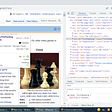# Report 3:

Before cropping you also need `haarcascade_frontalface_alt.xml` , which can be found here

`python retrain.py --output_graph=retrained_graph.pb --output_labels=retrained_labels.txt --architecture=MobileNet_1.0_224 --image_dir=images`
`python label.py`
`import cv2import label_imagesize = 4# We load the xml fileclassifier = cv2.CascadeClassifier('F:/dd/Library/etc/haarcascades/haarcascade_frontalface_alt.xml')im = cv2.imread('C:/Users/Faiz Khan/Desktop/ddd/facial expression/test/3.jpg', 0 )#im=cv2.flip(im,1,0) #Flip to act as a mirror# Resize the image to speed up detectionmini = cv2.resize(im, (int(im.shape/size), int(im.shape/size)))# detect MultiScale / faces faces = classifier.detectMultiScale(mini)# Draw rectangles around each facefor f in faces:    (x, y, w, h) = [v * size for v in f] #Scale the shapesize backup    cv2.rectangle(im, (x,y), (x+w,y+h), (0,255,0), 4)            #Save just the rectangle faces in SubRecFaces    sub_face = im[y:y+h, x:x+w]FaceFileName = "test.jpg" #Saving the current image for testing.    cv2.imwrite(FaceFileName, sub_face)            text = label_image.main(FaceFileName)# Getting the Result from the label_image file, i.e., Classification Result.    text = text.title()# Title Case looks Stunning.    font = cv2.FONT_HERSHEY_TRIPLEX    cv2.putText(im, text,(x,y), font, 1, (255,0,0), 2) # Show the imagecv2.imshow('Capture',   im)key = cv2.waitKey(10000)`
`from __future__ import absolute_importfrom __future__ import divisionfrom __future__ import print_functionimport argparseimport sysimport timeimport numpy as npimport tensorflow as tfdef load_graph(model_file):  graph = tf.Graph()  graph_def = tf.GraphDef()with open(model_file, "rb") as f:    graph_def.ParseFromString(f.read())  with graph.as_default():    tf.import_graph_def(graph_def)return graphdef read_tensor_from_image_file(file_name, input_height=299, input_width=299,    input_mean=0, input_std=255):  input_name = "file_reader"  output_name = "normalized"  file_reader = tf.read_file(file_name, input_name)  if file_name.endswith(".png"):    image_reader = tf.image.decode_png(file_reader, channels = 3,                                       name='png_reader')  elif file_name.endswith(".gif"):    image_reader = tf.squeeze(tf.image.decode_gif(file_reader,                                                  name='gif_reader'))  elif file_name.endswith(".bmp"):    image_reader = tf.image.decode_bmp(file_reader, name='bmp_reader')  else:    image_reader = tf.image.decode_jpeg(file_reader, channels = 3,                                        name='jpeg_reader')  float_caster = tf.cast(image_reader, tf.float32)  dims_expander = tf.expand_dims(float_caster, 0);  resized = tf.image.resize_bilinear(dims_expander, [input_height, input_width])  normalized = tf.divide(tf.subtract(resized, [input_mean]), [input_std])  sess = tf.Session()  result = sess.run(normalized)return resultdef load_labels(label_file):  label = []  proto_as_ascii_lines = tf.gfile.GFile(label_file).readlines()  for l in proto_as_ascii_lines:    label.append(l.rstrip())  return labeldef main(img):  file_name = img  model_file = "retrained_graph.pb"  label_file = "retrained_labels.txt"  input_height = 224  input_width = 224  input_mean = 128  input_std = 128  input_layer = "input"  output_layer = "final_result"parser = argparse.ArgumentParser()  parser.add_argument("--image", help="image to be processed")  parser.add_argument("--graph", help="graph/model to be executed")  parser.add_argument("--labels", help="name of file containing labels")  parser.add_argument("--input_height", type=int, help="input height")  parser.add_argument("--input_width", type=int, help="input width")  parser.add_argument("--input_mean", type=int, help="input mean")  parser.add_argument("--input_std", type=int, help="input std")  parser.add_argument("--input_layer", help="name of input layer")  parser.add_argument("--output_layer", help="name of output layer")  args = parser.parse_args()if args.graph:    model_file = args.graph  if args.image:    file_name = args.image  if args.labels:    label_file = args.labels  if args.input_height:    input_height = args.input_height  if args.input_width:    input_width = args.input_width  if args.input_mean:    input_mean = args.input_mean  if args.input_std:    input_std = args.input_std  if args.input_layer:    input_layer = args.input_layer  if args.output_layer:    output_layer = args.output_layergraph = load_graph(model_file)  t = read_tensor_from_image_file(file_name,                                  input_height=input_height,                                  input_width=input_width,                                  input_mean=input_mean,                                  input_std=input_std)input_name = "import/" + input_layer  output_name = "import/" + output_layer  input_operation = graph.get_operation_by_name(input_name);  output_operation = graph.get_operation_by_name(output_name);with tf.Session(graph=graph) as sess:    start = time.time()    results = sess.run(output_operation.outputs,                      {input_operation.outputs: t})    end=time.time()  results = np.squeeze(results)top_k = results.argsort()[-5:][::-1]  labels = load_labels(label_file)for i in top_k:    return labels[i]`
1. ) Here I have defined my flask app. I have also defined a static folder. All the files shown to the user is inside the templates folder.

--

--

--

## More from Faiz Khan

Love podcasts or audiobooks? Learn on the go with our new app.

## Deep Hunt — Issue #32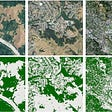## AI in travel, part 2: representing cyclic and geographic features## YOLOX: New Improved YOLO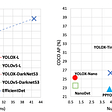## A review of BERT based models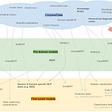## Extracting Colour Palettes with Unsupervised Learning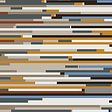## Faster Inference for NLP Pipeline’s using Hugging Face Transformers and ONNX Runtime## A deeper look into SqueezeDet on Keras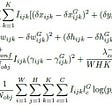## Wine Review PT5 — NLP — ML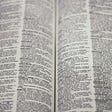## FULL-STACK APPLICATION FOR SPAM DETECTION## #Project | Pattern Recognition in Import Prices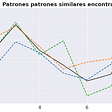## Introduction to Web Scraping -Simple Example